Problem: Evaluate (if it converges)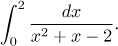Solution: If we fail to check on troubles, we would use integration by partial fractions decomposition and obtain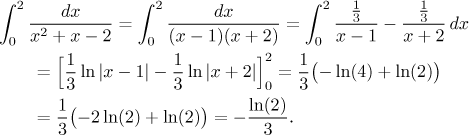This is, of course, wrong, because as we can see from the decomposition, there are two points where the denominator is zero. Unfortunately, one of them, namely x = 1, is in the middle of the integration interval, so the above evaluation cannot be used. We have to split the given integral into two basic improper integrals: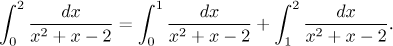Since we already determined an antiderivative, we can immediately start evaluating the basic improper integrals; as noted before, it is vital to put the two logarithms together.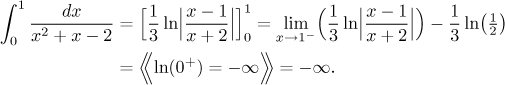Because the first part ends up divergent, the whole given integral diverges.

Does the given integral exist? To answer that we need to evaluate also its other part.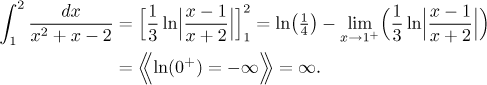Resulting infinities have opposite signs, so when we add both parts of the given integral, we get the indeterminate expression ∞ − ∞. Thus the given integral does not exist.